Test: Feedback Amplifier

# Test: Feedback Amplifier

Test Description

## 15 Questions MCQ Test GATE Electrical Engineering (EE) 2023 Mock Test Series | Test: Feedback Amplifier

Test: Feedback Amplifier for Electrical Engineering (EE) 2023 is part of GATE Electrical Engineering (EE) 2023 Mock Test Series preparation. The Test: Feedback Amplifier questions and answers have been prepared according to the Electrical Engineering (EE) exam syllabus.The Test: Feedback Amplifier MCQs are made for Electrical Engineering (EE) 2023 Exam. Find important definitions, questions, notes, meanings, examples, exercises, MCQs and online tests for Test: Feedback Amplifier below.
Solutions of Test: Feedback Amplifier questions in English are available as part of our GATE Electrical Engineering (EE) 2023 Mock Test Series for Electrical Engineering (EE) & Test: Feedback Amplifier solutions in Hindi for GATE Electrical Engineering (EE) 2023 Mock Test Series course. Download more important topics, notes, lectures and mock test series for Electrical Engineering (EE) Exam by signing up for free. Attempt Test: Feedback Amplifier | 15 questions in 45 minutes | Mock test for Electrical Engineering (EE) preparation | Free important questions MCQ to study GATE Electrical Engineering (EE) 2023 Mock Test Series for Electrical Engineering (EE) Exam | Download free PDF with solutions
 1 Crore+ students have signed up on EduRev. Have you?
Test: Feedback Amplifier - Question 1

### The negative feedback in an amplifier leads to which one of the following?

Detailed Solution for Test: Feedback Amplifier - Question 1

The negative feedback in amplifiers causes:

• Reduced the gain and increases the stability in G.
• Increases the bandwidth to maintain constant gain-bandwidth product
• Reduces the distortion and noise in the amplifier
• The signal-to-noise ratio is not affected.
• The voltage gain (Av) of an amplifier is defined as the ratio of output voltage to the input voltage.

Av = Vo/Vin

Here, Vo is the output voltage of an amplifier and Vin is the input voltage of an amplifier.

• In a negative feedback amplifier, closed-loop voltage gain is given

Av = Vo / Vin = 1/(1+Aoβ)

Here, β = feedback factor,

Ao = open-loop gain of the amplifier.

• This expression clearly shows that closed-loop voltage gain has reduced by introducing negative feedback.
• We know that a product of gain and bandwidth is inversely proportional so here bandwidth of amplifier will increase ;

(gain × bandwidth = 0.35)

• A negative feedback amplifier decreases the current gain.
Test: Feedback Amplifier - Question 2

### Applications of negative feedback to a certain amplifier reduced its gain from 200 to 100. If the gain with the same feedback is to be raised to 150, in the case of another such appliance, the gain of the amplifier without feedback must have been

Detailed Solution for Test: Feedback Amplifier - Question 2

Concept:

A negative feedback network is shown below.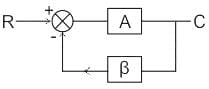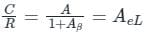Where A is the gain of the amplifier without feedback and feedback gain = β

Calculation:

Case 1:

Gain without feedback, A = 200

Gain with feedback ACL = 100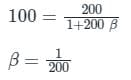Case 2:

Gain with feedback, ACL = 150

Feedback,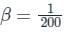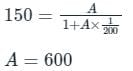Test: Feedback Amplifier - Question 3

### An amplifier has a Open Loop voltage gain of –500. This gain is reduced to –100 when negative feedback is applied. The reverse transmission factor,β of this system is:-

Detailed Solution for Test: Feedback Amplifier - Question 3

Concept:

The gain of a feedback system is given by: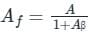A = Open Loop gain

Af = Closed Loop Gain

β = Feedback/Transmission factor

Calculation:

Given Af = -100 and A = -500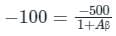1 + Aβ = 5

Aβ = 4

β = 4 / -500 = -0.008

Test: Feedback Amplifier - Question 4

The feedback topology in the amplifier circuit (the base bias circuit is not shown for simplicity) in the figure is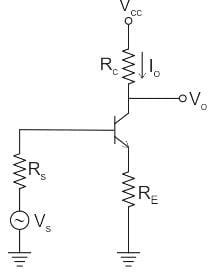Detailed Solution for Test: Feedback Amplifier - Question 4

In a current series feedback, current is sampled from the output and voltage is feedback to the source.

In the given amplifier circuit, the feedback signal becomes zero by opening the output feedback.

Hence, it is a current series feedback.

*Answer can only contain numeric values
Test: Feedback Amplifier - Question 5

It is desired to reduce total harmonic of amplifier from 8% to 1% by use of 10% negative feedback. If the gain of the amplifier with original distortion and with reduced distortion is A1 and A2 then, A1 + A2 = −−−−−−

Detailed Solution for Test: Feedback Amplifier - Question 5

Given that β = 0.1and we know that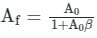So we can write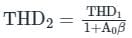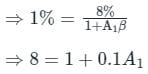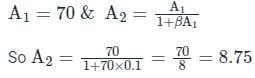∴ A1 + A2 =78.75

Test: Feedback Amplifier - Question 6

Which of these doesn’t refer to a series-shunt feedback?

Detailed Solution for Test: Feedback Amplifier - Question 6

In a series shunt feedback network, feedback is connected in series with signal source but in shunt with the load. Error voltage from feedback network is in series with the input. Voltage fed back from output is proportional to output voltage, hence parallel or shunt connected. The current in and voltage out connection refers to a shunt-shunt connection.

Test: Feedback Amplifier - Question 7

Consider a voltage series feedback network, where amplifier gain = 100, feedback factor = 5. For the basic amplifier, input voltage = 4V, input current=2mA. Find the input resistance of the network.

Detailed Solution for Test: Feedback Amplifier - Question 7

RI = VI/II = 4/2m = 2kΩ
RIF = RI(1+A.β) = 2k(1+500)
= 1002kΩ.

Test: Feedback Amplifier - Question 8

In which network is the unit of the feedback factor Ω?

Detailed Solution for Test: Feedback Amplifier - Question 8

In series-series feedback, the output is current sampled, that is it is in series with the load. Also, input is a voltage mixer, which is in series with signal source. So feedback factor
Β = VF/IL in Ohms.

Test: Feedback Amplifier - Question 9

Consider given circuit.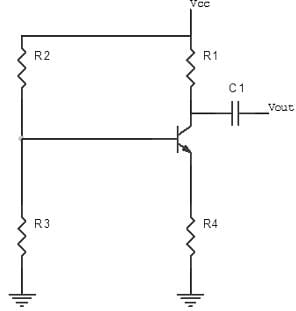What is the feedback configuration?

Detailed Solution for Test: Feedback Amplifier - Question 9

The resistance R4 is the feedback network resistance. There is no bypass capacitor being used. The resistance is not directly connected to either the input node or output node. Hence it’s a current series feedback.

Test: Feedback Amplifier - Question 10

In a feedback network, input voltage is 14V, feedback voltage is 6V and source voltage is 20V. β is in ohms. What is its configuration?

Detailed Solution for Test: Feedback Amplifier - Question 10

Given that input is 14V, feedback is 6V and source is 20 V, we can see
VI = VS – VF, which is voltage mixing. Also, β is in ohms that is voltage/current.
Since output of feedback is voltage and input is current, the output has current sampling.
Thus, configuration is a series-series feedback/current – series feedback.

Test: Feedback Amplifier - Question 11

Find the relative change in gain with negative feedback given that return ratio is 24, and feedback factor is 3, when the change in open loop gain is 2.

Detailed Solution for Test: Feedback Amplifier - Question 11

F = A/(1+Aβ)
Aβ = 24
A = 8
Relative change in gain = dAF/AF = dA/A(1+Aβ)
dAF/AF = 2/8*25 = 0.01.

Test: Feedback Amplifier - Question 12

What is the reverse transmission factor?

Detailed Solution for Test: Feedback Amplifier - Question 12

In feedback systems, the feedback signal is in proportion with the output signal.
XF ∝ XO
XF = βXO, where β is the feedback factor or reverse transmission factor.

Test: Feedback Amplifier - Question 13

Which factor determines the gain of the voltage series feedback amplifier?

Detailed Solution for Test: Feedback Amplifier - Question 13

In setting the gain of the voltage series feedback amplifier, the ratio of two resistors is important and not the absolute value of these resistors. For example: If a gain of 11 is desired, we choose R1 = 1kΩ and R1 = 10kΩ or R1 = 100Ω and RF = 1kΩ.

Test: Feedback Amplifier - Question 14

Voltage shunt feedback amplifier forms

Detailed Solution for Test: Feedback Amplifier - Question 14

A voltage shunt feedback amplifier forms a negative feedback because, any increase in the output signal results in a feedback signal into the inverting input causing a decrease in the output signal.

Test: Feedback Amplifier - Question 15

Which among the following is not a special case of voltage shut feedback amplifier?

Detailed Solution for Test: Feedback Amplifier - Question 15

A voltage follower is a special case of non-inverting amplifier ( or voltage series feedback amplifier) and it has a gain of unity.

## GATE Electrical Engineering (EE) 2023 Mock Test Series

21 docs|273 tests
 Use Code STAYHOME200 and get INR 200 additional OFF Use Coupon Code
Information about Test: Feedback Amplifier Page
In this test you can find the Exam questions for Test: Feedback Amplifier solved & explained in the simplest way possible. Besides giving Questions and answers for Test: Feedback Amplifier, EduRev gives you an ample number of Online tests for practice

## GATE Electrical Engineering (EE) 2023 Mock Test Series

21 docs|273 tests